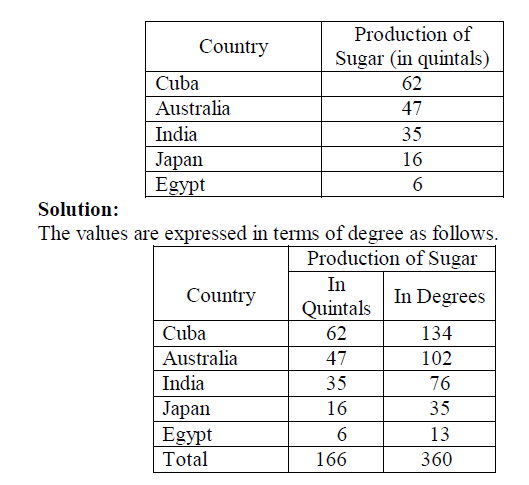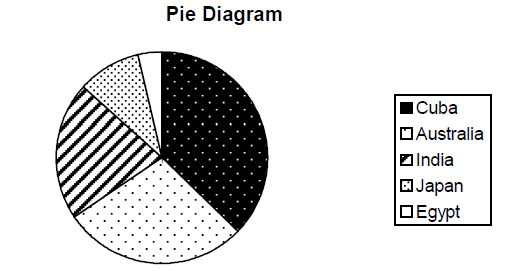# Statistics for SSC-CGL Tier-II (Paper-III) – Part-9

By | November 20, 2016

DIAGRAMATIC AND GRAPHICAL REPRESENTATION:

TYPES OF DIAGRAMS:

Two-dimensional Diagrams:

In one-dimensional diagrams, only length 9 is taken into account. But in two-dimensional diagrams the area represent the data and so the length and breadth have both to be taken into account. Such diagrams are also called area diagrams or surface diagrams.

The important types of area diagrams are:

1. Rectangles 2. Squares 3. Pie-diagrams

1.Rectangles:

Rectangles are used to represent the relative magnitude of two or more values. The area of the rectangle is kept in proportion to the values. Rectangles are placed side by side for comparison. When two sets of figures are to be represented by rectangles, either of the two methods may be adopted.

We may represent the figures as they are given or may convert them to percentages and then subdivide the length into various components. Thus the percentage sub-divided rectangular diagram is more popular than sub-divided rectangular since it enables comparison to be made on a percentage basis.

Example 6:

Represent the following data by sub-divided percentage rectangular diagram.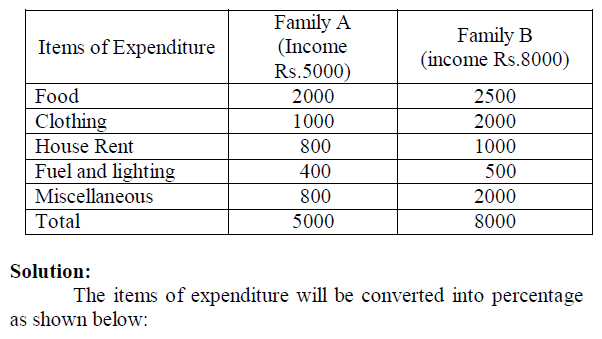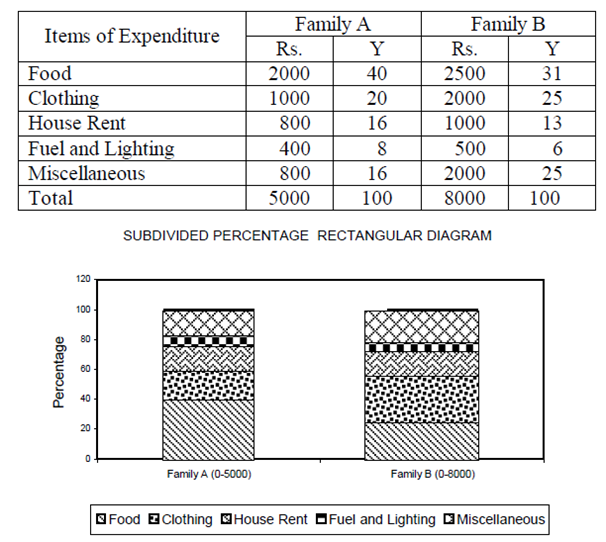2. Squares:

The rectangular method of diagrammatic presentation is difficult to use where the values of items vary widely. The method of drawing a square diagram is very simple. One has to take the square root of the values of various item that are to be shown in the diagrams and then select a suitable scale to draw the squares.

Example 7:

Yield of rice in Kgs. per acre of five countries are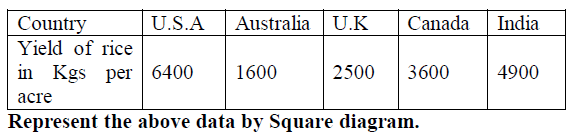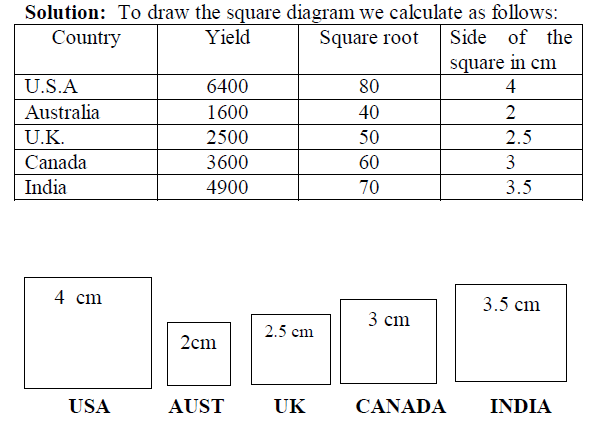3. Pie Diagram or Circular Diagram:

Another way of preparing a two-dimensional diagram is in the form of circles. In such diagrams, both the total and the component parts or sectors can be shown. The area of a circle is proportional to the square of its radius.

While making comparisons, pie diagrams should be used on a percentage basis and not on an absolute basis. In constructing a pie diagram the first step is to prepare the data so that various components values can be transposed into corresponding degrees on the circle.

The second step is to draw a circle of appropriate size with a compass. The size of the radius depends upon the available space and other factors of presentation. The third step is to measure points on the circle and representing the size of each sector with the help of a protractor.

Example 8:

Draw a Pie diagram for the following data of production of sugar in quintals of various countries.